# PV Solar Angles

Published: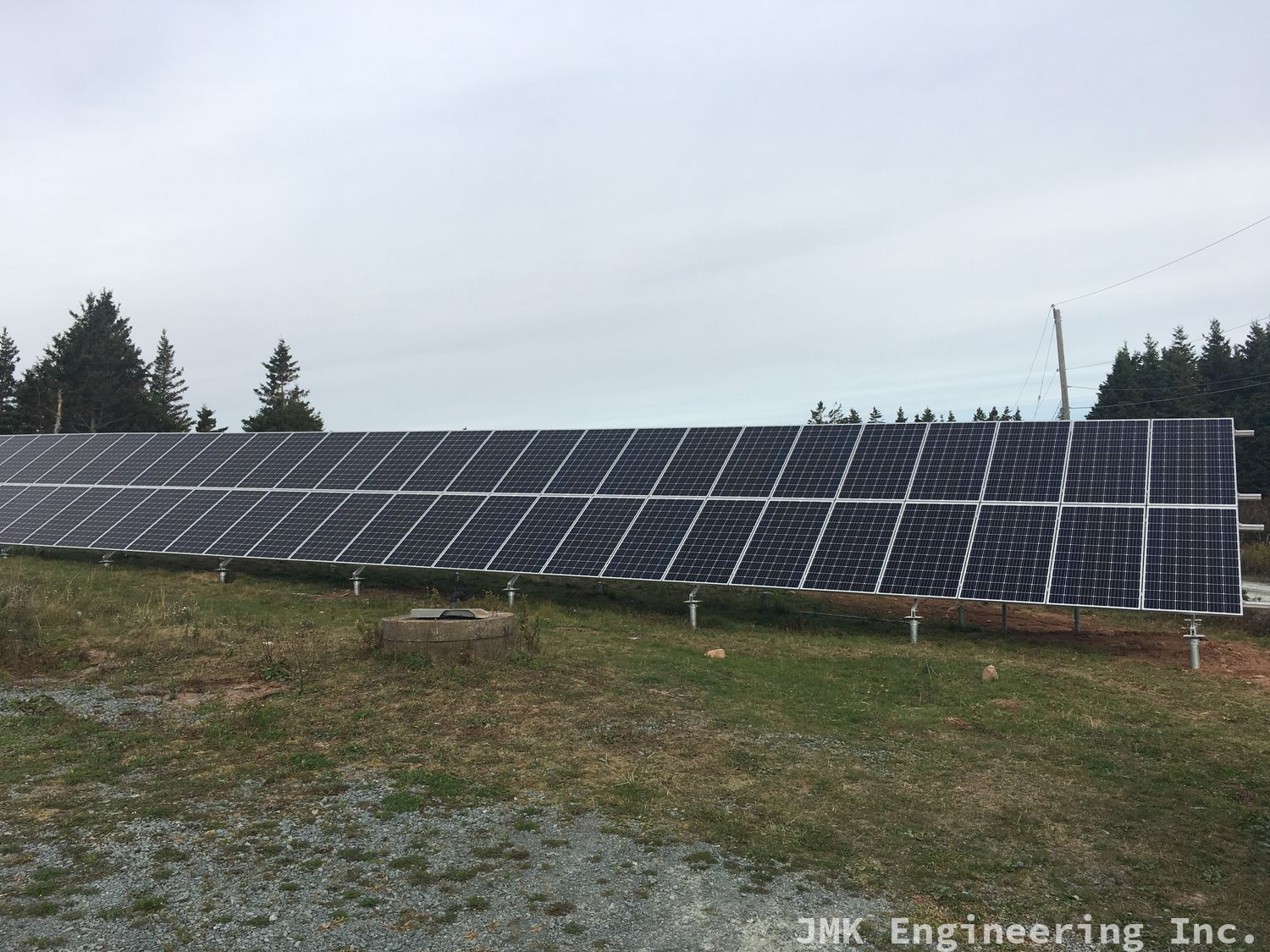Solar panels are most effective when the solar rays are perpendicular to the panel service. That's the first angle that matters, getting the panel pointing the right direction take a little bit of math.

The vast majority of the time you will use software that does this automatically, but doing a few by hand to understand what the software is doing is very helpful in getting a basic understanding of what's going on.

## So, what angles matter?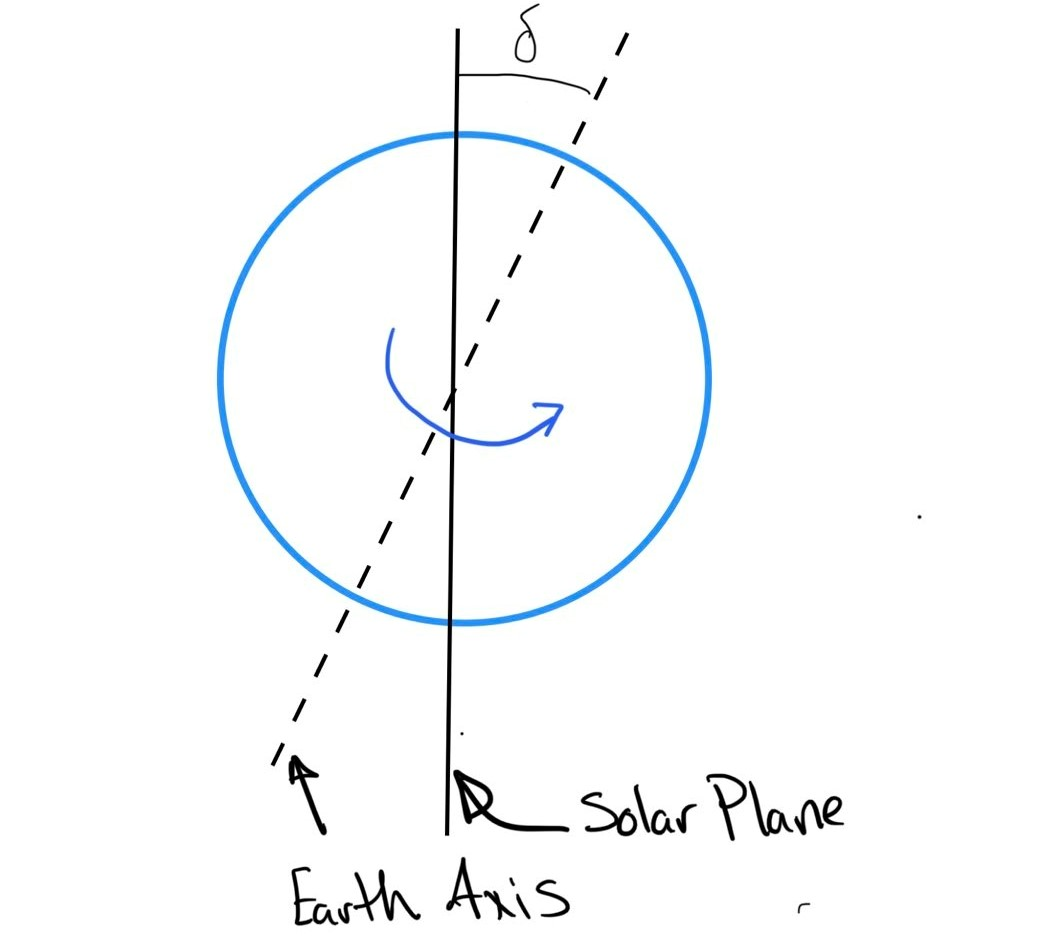Sketch showing declination angle

First angle we need to determine is the angle of declination (δ), this is the angle between the earth axis and the solar plane. It varies over the year and is calculated as:

δ  = 23.5*sin((360(284 + d))/(365))

where:

• d = day of the year

With δ calculated we can calculate the elevation angle α, this is the angle that the sun hits the earth during peak hours, solar noon.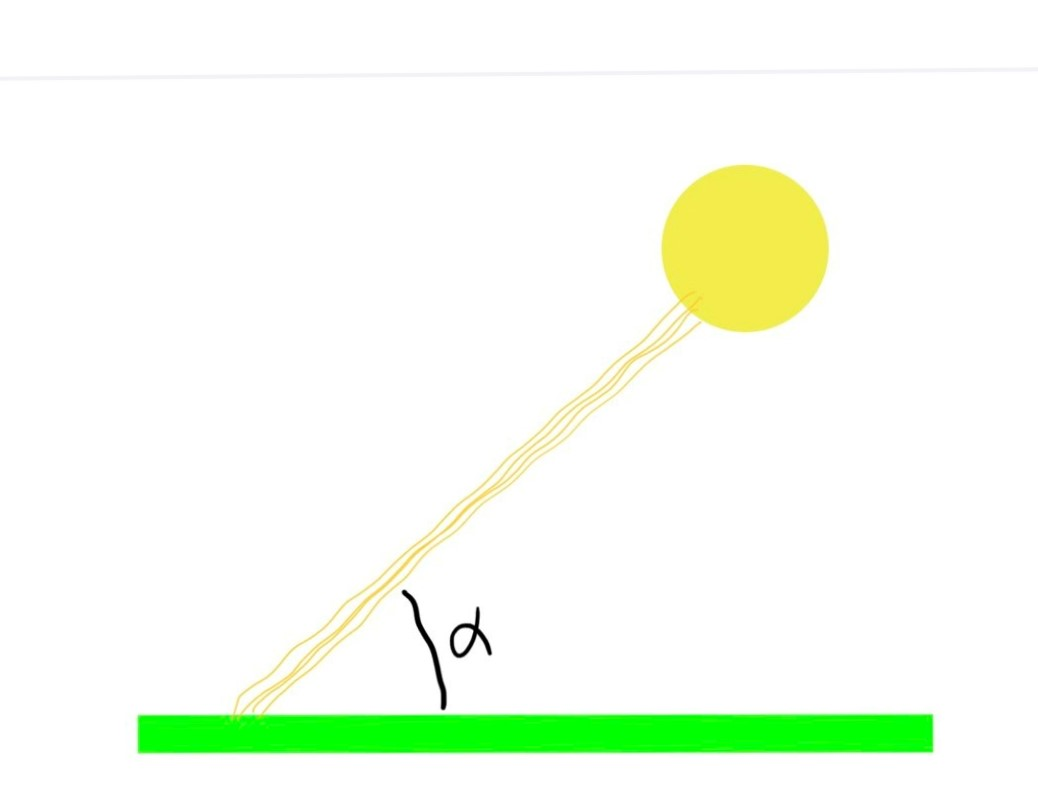Sketch showing elevation angle

α = ϕ + δ

where:

• ϕ = latitude

Because the highest irradience is at solar noon, for static systems we want to ensure that the panel is perpendicular to the sun at that time, that is where the elevation angle β comes in.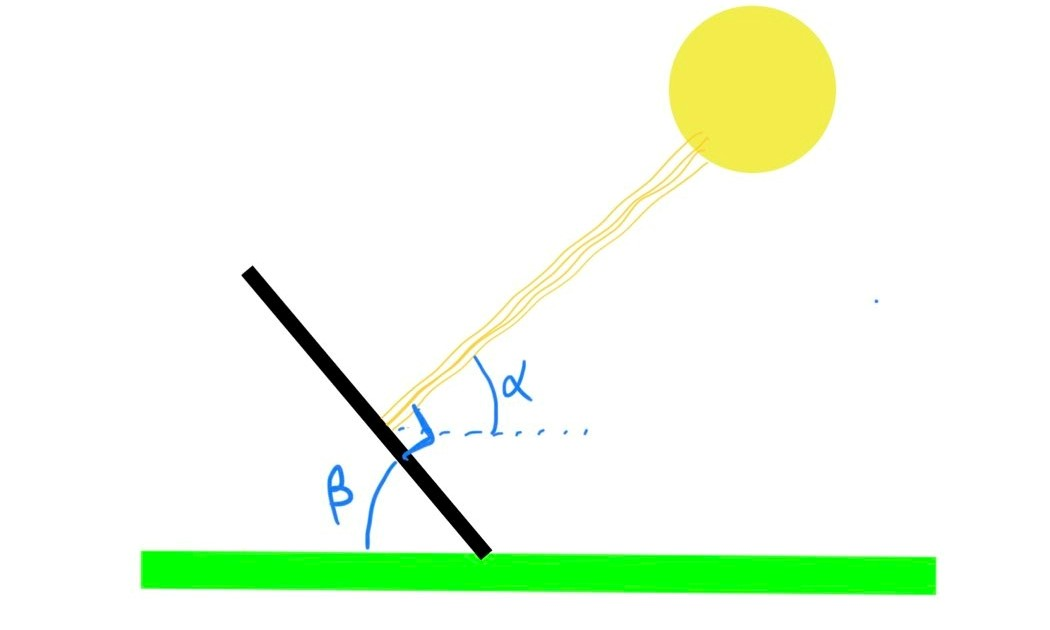Sketch showing the tilt angle

β = 90 − α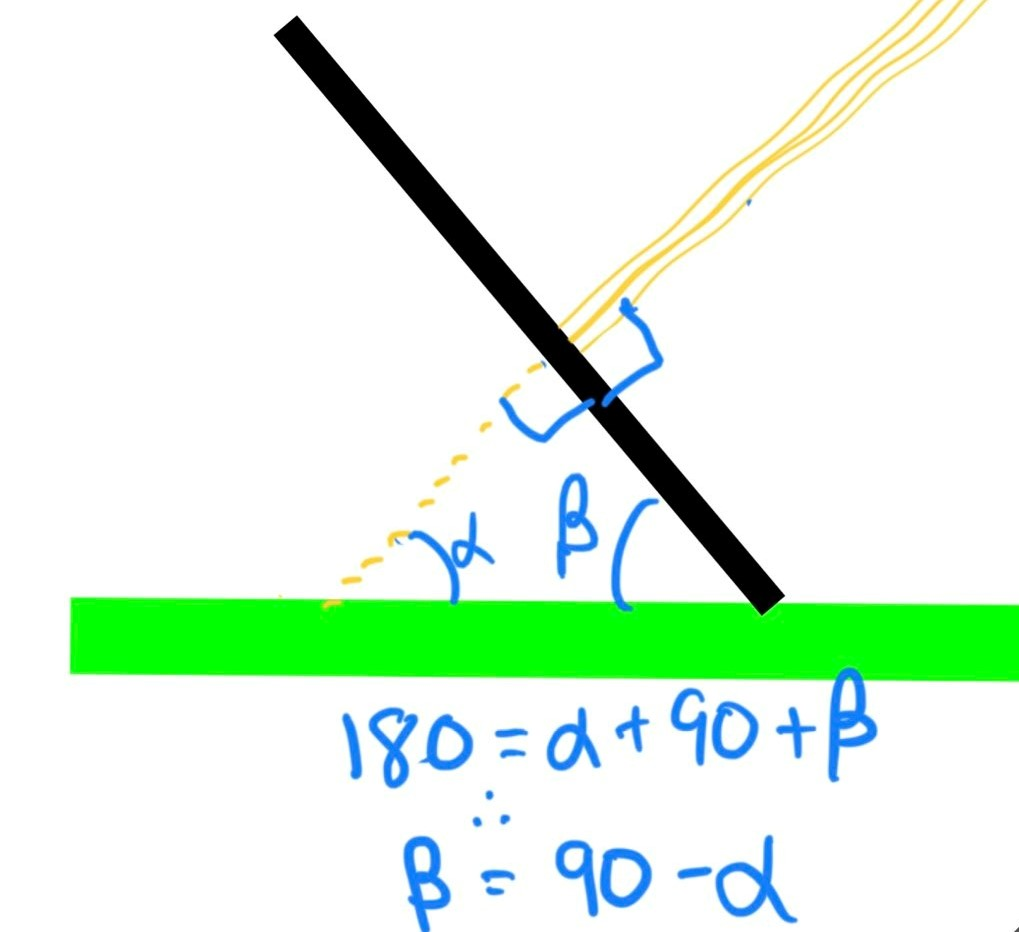Sketch showing how the tilt angle is calculated

β will always vary between 0 and 90 degrees, 90 closer to the poles and 0 at the equater.

## Next Steps

Once we have a handle of the various angles involved we need to start defining the various solar radiation and how it will determine the solar energy converted to electrical energy.

From there we can determine the optimal angle β for the panels, and how changing that angle will affect energy production.

This post is part 2 of the "PV Solar System Design" series: